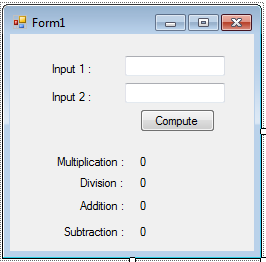# Arithmetic Operators

In programming, the Mathematical operations are very useful because most of the time we are dealing with mathematical concepts in programming. We know that computers can perform a mathematical calculation much faster than humans. Also the computer doesn’t have the capacity to do calculations without receiving any instructions from the computer user. Using Visual Basic, we are able to give instructions to the computer to perform mathematical operations such as Multiplication, Division, Addition, Subtraction and other kinds of arithmetic operations. List of Arithmetic Operators in Visual Basic.
```Sysmbol	Name
*	Multiplication
/	Division
-	Subtraction
\	Integer Division
Mod	Modolo
^	Exponentiation
```
This time, we’re going to create a program performing the Arithmetic Operators. To do this, open Visual Basic and create a new project then save it as “Arithmetic Operators”. Next, add two textbox, six labels and a button. Deigning the Object Properties.
```Object		Property		Settings
Textbox1	Name			txtinput1
Textbox2	Name			txtinput2
Label1		Name			lblmul
Text			0
Label2		Name			lbldiv
Text			0
Text			0
Label1		Name			llblsub
Text			0
Button1	        Name			btncompute
Text			Compute
```
Then,arrange all object looks like as shown below.At this time, we will add functionality to our application. To do this, double click the” Compute” button and add the following code:
`Dim input1 As IntegerDim input2 As Integer input1 = txtinput1.Text ' assign value to a variableinput2 = txtinput2.Text lblmul.Text = input1 * input2 ' compute for Multiplicationlbldiv.Text = input1 / input2 ' compute for Divisionlbladd.Text = input1 + input2 ' compute for Additionlblsub.Text = input1 - input2 ' compute for Subttraction`
After adding the code above, you can now test your application by pressing “F5”.